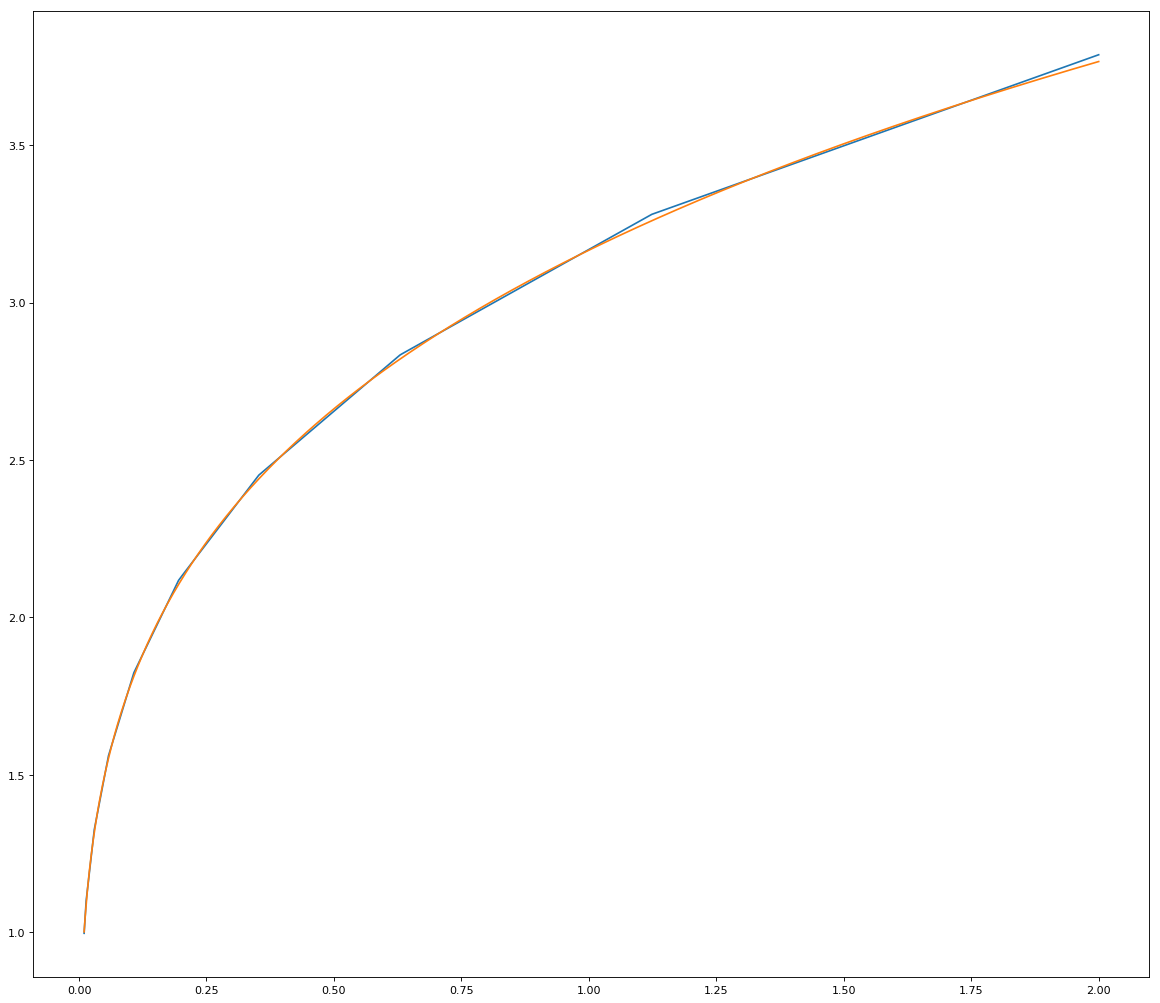# Applying the Finite Element Method to the Deterministic Growth Model

Suppose we want to solve the following problem by using the finite element method.

$$\max_{\{c_t\}_{t=0}^\infty} \sum_{t=0}^\infty \beta^t \log(c_t)$$ subject to $$c_t + k_{t+1} - (1-\delta) k_t = Ak_t^\theta$$

Why do we want to use the finite element method?

We could write the problem recursively, and then use the value function iteration method to solve for the optimal path. However, it wouldn't give us the consumption as a function of any capital level $k$; what we get would be the consumption levels for several values of $k$ in the grid.

By using the finite element method, we approximate our consumption function to a linear combination of piecewise linear functions, which is computationally much less costly compared to the value function iteration method.

Following McGrattan (1999), I set up the model as follows:

• We set $\beta = 0.96$, $\theta = 0.25$, $A = 1/(\beta\theta)$, and $\delta = 1$ (as a test case).
In :
import numpy as np
from numpy import linalg
import matplotlib.pyplot as plt
import scipy
from scipy import optimize

beta = 0.96;
theta = 0.25;
delta = 1;
A = 1/(theta*beta);

• 10 elements
• 11 distinct boundary points: $$x_0 = 0, x_{10} = 2, x_i = x_{i-1} + 0.005 \exp(0.574(i - 2))$$
In :
n = 10;
def setupIntervalLimits(n):
k_init = 0;
k_end = 2;
k = np.zeros((n,));
k = k_init;
for i in range(1,n):
k[i] = k[i-1] + 0.005 * np.exp(0.574*(i-1));
k[-1] = k_end;
return k;

• Legendre quadrature to calculate weighted integral
• Two quadrature points for each element $e\in \{0,1,\ldots,9\}$ $$w = l_e/2$$ $$k_{1e} = k_e + 0.211l_e,\;\; k_{2e} = k_e +0.789l_e$$
In :
def elementLengths(k):
l = np.zeros((len(k)-1,));
for i in range(len(l)):
l[i] = k[i+1]-k[i];
return l;

l = elementLengths(k);
w = l/2;

k1 = np.zeros((n,));
k2 = np.zeros((n,));

for e in range(n):
k1[e] = k[e] + l[e] * 0.211;
k2[e] = k[e] + l[e] * 0.789;

return w, k1, k2;

k_vec = setupIntervalLimits(n+1);

• Construct $\psi_i(k)$ as $$\psi_i(k) = \left\{ \begin{array}{cl} \frac{k-k_{i-1}}{k_i - k_{i-1}} & \text{if } k\in [k_{i-1}, k_i] \\ \frac{k_{i+1}-k}{k_{i+1} - k_i} & \text{if } k\in [k_{i}, k_{i+1}] \\ 0 & \text{otherwise} \end{array}\right.$$
In :
def findPsi(i, k, k_vec):
if(i<n):
if(k >= k_vec[i-1] and k<= k_vec[i]):
p = (k-k_vec[i-1])/(k_vec[i]-k_vec[i-1]);
elif(k >= k_vec[i] and k <= k_vec[i+1]):
p = (k_vec[i+1]-k)/(k_vec[i+1]-k_vec[i]);
else:
p = 0;
elif(i==n):
if(k >= k_vec[i-1] and k<= k_vec[i]):
p = (k-k_vec[i-1])/(k_vec[i]-k_vec[i-1]);
else:
p = 0;
return p;

• Construct the residual function. To do that, we solve for the Euler equation for our model.

$$[c_t]: \frac{\beta^t}{c_t} - \lambda_t = 0 [k_{t+1}]: -\lambda_t + \lambda_{t+1}[A\theta k_{t+1}^{\theta-1} + (1-\delta)] = 0 [\lambda_t] : c_t + k_{t+1} - (1-\delta)k_t = Ak_t^\theta$$

Therefore, we get \begin{align*} c_{t+1} * = \beta c_t [A\theta k_{t+1}^{\theta-1} + 1-\delta]\\ k_{t+1} = (1-\delta)k_t + Ak_t^\theta - c_{t} \end{align*}

Transforming the equations to a recursive notation, and writing $c$ as a function of the current state $k$, we get

\begin{align*} c(k') = \beta c(k) [A\theta (k')^{\theta-1} + 1-\delta]\\ k' = (1-\delta)k + Ak^\theta - c(k) \end{align*}

We can write the Euler equation as:

$$\frac{\beta c(k) [A\theta (k')^{\theta-1} + 1-\delta]}{c(k')} - 1 = 0$$

Replacing $k'$ into this equation, we get

$$\frac{\beta c(k) [A\theta (Ak^\theta + (1-\delta)k - c(k))^{\theta-1} + 1-\delta]}{c(Ak^\theta + (1-\delta)k - c(k))} - 1 = 0$$

Note that we approximate $c(k)$ as

$$c^n(k) = \sum_{i=0}^{10} \alpha_i \psi_i(k)$$

So, we'll use this approximation to calculate

$$R(c^n)(k) = \frac{\beta c^n(k) [A\theta (Ak^\theta + (1-\delta)k - c^n(k))^{\theta-1} + 1-\delta]}{c^n(Ak^\theta + (1-\delta)k - c^n(k))} - 1$$

In :
def findR(k, alpha):
d = 0;
for i in range(n+1):
d += alpha[i] * findPsi(i, k, k_vec);
if(A*(k**theta)+(1-delta)*k<d or d<0 or A*(k**theta)-d+(1-delta)*k>2):
if(d<0):
return (punish*d);
elif(A*(k**theta)<d):
return abs(punish*(A*(k**theta)-d+(1-delta)*k));
else:
return (punish*(2-A*(k**theta) + d-(1-delta)*k));
else:
nom = beta*d*(A*theta*np.power((A*(k**theta) + (1-delta)*k - d), theta-1)+1-delta);
denom = 0;
for i in range(n+1):
denom += alpha[i] * findPsi(i,A*(k**theta)+(1-delta)*k-d,k_vec);
else:
return nom/denom - 1;


Finally, what we want is to find $\alpha\equiv [\alpha_0,\alpha_1,\ldots,\alpha_{10}]$ that solves

$$\int \psi_i(k)R(k,\alpha)dk = 0 \;\;, \;\; i=0,1,\ldots,10$$

To numerically calculate the integral, we use the Legendre quadrature weights and points that we set up earlier. So, our equation becomes:

$$\sum_{j=0}^9 \left\{w_{1j}\psi_i(k_{1j})R(k_{1j},\alpha) + w_{2j}\psi_i(k_{2j})R(k_{2j},\alpha)\right\} = 0 \;\;, \;\; i=0,1,\ldots,10$$

In :
def sysOfEqns(alpha):

eqn = np.zeros((n+1,));
for i in range(n+1):
temp = 0;
for j in range(n):
R1 = findR(k1[j],alpha);
R2 = findR(k2[j],alpha);
temp += w[j]*findPsi(i,k1[j],k_vec)*R1 + w[j]*findPsi(i,k2[j],k_vec)*R2;
eqn[i] = temp;
return eqn;


To satisfy the boundary condition, we set $\alpha_0 = 0$. We also drop the first ($i=0$) equation. Therefore, we have 10 equations and 10 unknown $\alpha_i$'s ($i=1,\ldots,10$). Hence, we can find the root of the system of equations.

In :
punish = 60000;
text = '';

init_alpha = np.array([1,-.23,1,1,1,1,1,1,1,1,1]);
while(text != 'The solution converged.'):
punish = punish / 2;
u = scipy.optimize.root(sysOfEqns, init_alpha, tol=1e-12);
text = u.message;
alpha = u.x;


Once we get the $\alpha$ values, we can find the approximation for the consumption function $c^n(k)$.

In :
def findCons(k):
c = 0;
for i in range(1,n+1):
c += alpha[i] * findPsi(i, k, k_vec);
return c;


Finally, let's plot the consumption vs. capital and see how successful we matched the theoretical solution. To do that, we use $\delta=1$ in order to get the theoretical solution $c = (1-\theta\beta)*A*k^\theta$.

In :
fig=plt.figure(figsize=(18, 16), dpi= 80, facecolor='w', edgecolor='k')

N = 1000;
my_k = np.linspace(0.01,2,N)
my_c = np.zeros((N,))
th_c = np.zeros((N,))

for i in range(N):
my_c[i] = findCons(my_k[i]);
if(delta==1):
th_c[i] = (1-theta*beta)*(A*my_k[i]**theta);

plt.plot(my_k,my_c)
if(delta==1):
plt.plot(my_k,th_c)

plt.show()References:

McGrattan, Ellen (1999). "Application of Weighted Residual Methods to Dynamic Economic Models" in Computational Methods for the Study of Dynamic Economies, ed. Ramon Marimon and Andrew Scott.